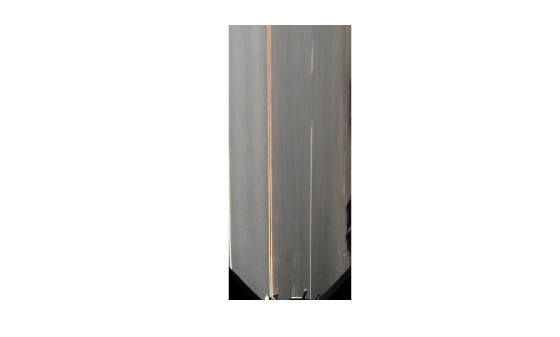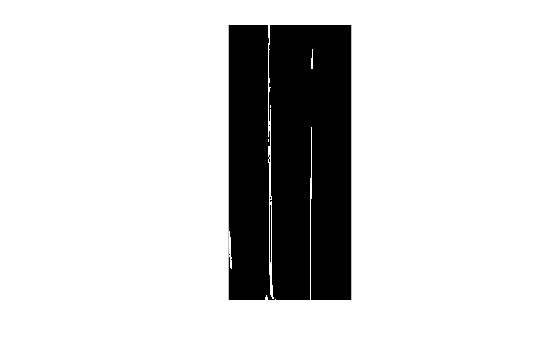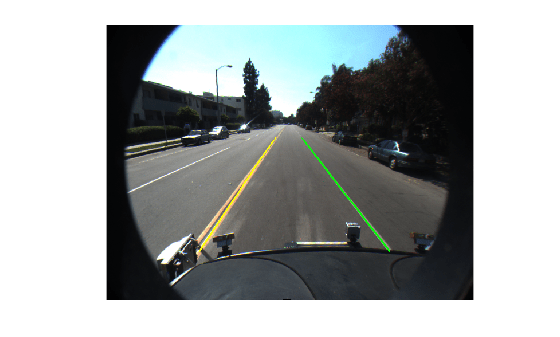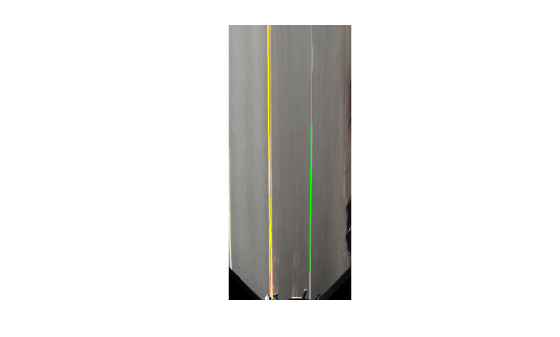# findCubicLaneBoundaries

Find boundaries using cubic model

## Syntax

``boundaries = findCubicLaneBoundaries(xyBoundaryPoints,approxBoundaryWidth)``
``````[boundaries,boundaryPoints] = findCubicLaneBoundaries(xyBoundaryPoints,approxBoundaryWidth)``````
``[___] = findCubicLaneBoundaries(___,Name,Value)``

## Description

example

````boundaries = findCubicLaneBoundaries(xyBoundaryPoints,approxBoundaryWidth)` uses the random sample consensus (RANSAC) algorithm to find cubic lane boundary models that fit a set of boundary points and an approximate width. Each model in the returned array of `cubicLaneBoundary` objects contains the ```[A B C D]``` coefficients of its third-degree polynomial equation and the strength of the boundary estimate.```
``````[boundaries,boundaryPoints] = findCubicLaneBoundaries(xyBoundaryPoints,approxBoundaryWidth)``` also returns a cell array of inlier boundary points for each boundary model found, using the previous input arguments.```
````[___] = findCubicLaneBoundaries(___,Name,Value)` uses options specified by one or more `Name,Value` pair arguments, with any of the preceding syntaxes.```

## Examples

collapse all

Find lanes in an image by using cubic lane boundary models. Overlay the identified lanes on the original image and on a bird's-eye-view transformation of the image.

Load an image of a road with lanes. The image was obtained from a camera sensor mounted on the front of a vehicle.

`I = imread('road.png');`

Transform the image into a bird's-eye-view image by using a preconfigured sensor object. This object models the sensor that captured the original image.

```bevSensor = load('birdsEyeConfig'); birdsEyeImage = transformImage(bevSensor.birdsEyeConfig,I); imshow(birdsEyeImage)```Set the approximate lane marker width in world units (meters).

`approxBoundaryWidth = 0.25;`

Detect lane features and display them as a black-and-white image.

```birdsEyeBW = segmentLaneMarkerRidge(im2gray(birdsEyeImage), ... bevSensor.birdsEyeConfig,approxBoundaryWidth); imshow(birdsEyeBW)```Obtain lane candidate points in world coordinates.

```[imageX,imageY] = find(birdsEyeBW); xyBoundaryPoints = imageToVehicle(bevSensor.birdsEyeConfig,[imageY,imageX]);```

Find lane boundaries in the image by using the `findCubicLaneBoundaries` function. By default, the function returns a maximum of two lane boundaries. The boundaries are stored in an array of `cubicLaneBoundary` objects.

`boundaries = findCubicLaneBoundaries(xyBoundaryPoints,approxBoundaryWidth);`

Use `insertLaneBoundary` to overlay the lanes on the original image. The `XPoints` vector represents the lane points, in meters, that are within range of the ego vehicle's sensor. Specify the lanes in different colors. By default, lanes are yellow.

```XPoints = 3:30; figure sensor = bevSensor.birdsEyeConfig.Sensor; lanesI = insertLaneBoundary(I,boundaries(1),sensor,XPoints); lanesI = insertLaneBoundary(lanesI,boundaries(2),sensor,XPoints,'Color','green'); imshow(lanesI)```View the lanes in the bird's-eye-view image.

```figure BEconfig = bevSensor.birdsEyeConfig; lanesBEI = insertLaneBoundary(birdsEyeImage,boundaries(1),BEconfig,XPoints); lanesBEI = insertLaneBoundary(lanesBEI,boundaries(2),BEconfig,XPoints,'Color','green'); imshow(lanesBEI)```## Input Arguments

collapse all

Candidate boundary points, specified as an `[x y]` vector in vehicle coordinates. To obtain the vehicle coordinates for points in a `birdsEyeView` image, use the `imageToVehicle` function to convert the bird's-eye-view image coordinates to vehicle coordinates.

Approximate boundary width, specified as a real scalar in world units. The width is a horizontal y-axis measurement.

### Name-Value Pair Arguments

Specify optional comma-separated pairs of `Name,Value` arguments. `Name` is the argument name and `Value` is the corresponding value. `Name` must appear inside quotes. You can specify several name and value pair arguments in any order as `Name1,Value1,...,NameN,ValueN`.

Example: `'MaxSamplingAttempts',200`

Maximum number of lane boundaries that the function attempts to find, specified as the comma-separated pair consisting of `'MaxNumBoundaries'` and a positive integer.

Function to validate the boundary model, specified as the comma-separated pair consisting of `'ValidateBoundaryFcn'` and a function handle. The specified function returns logical `1` (true) if the boundary model is accepted and logical `0` (false) otherwise. Use this function to reject invalid boundaries. The function must be of the form:

`isValid = validateBoundaryFcn(parameters)`

`parameters` is a vector corresponding to the three parabolic parameters.

The default validation function always returns `1` (true).

Maximum number of attempts to find a sample of points that yields a valid cubic boundary, specified as the comma-separated pair consisting of `'MaxSamplingAttempts'` and a function handle. `findCubicLaneBoundaries` uses the `fitPolynomialRANSAC` function to sample from the set of boundary points and fit a cubic boundary line.

## Output Arguments

collapse all

Lane boundary models, returned as an array of `cubicLaneBoundary` objects. Lane boundary objects contain the following properties:

• `Parameters` — A four-element vector, `[A B C D]`, that corresponds to the four coefficients of a third-degree polynomial equation in general form: y = Ax3 + Bx2 + Cx + D.

• `BoundaryType` — A `LaneBoundaryType` of supported lane boundaries. The supported lane boundary types are:

• `Unmarked`

• `Solid`

• `Dashed`

• `BottsDots`

• `DoubleSolid`

Specify a lane boundary type as `LaneBoundaryType.BoundaryType`. For example:

```LaneBoundaryType.BottsDots ```
• `Strength` — A ratio of the number of unique x-axis locations on the boundary to the total number of points along the line, based on the `XExtent` property.

• `XExtent` — A two-element vector describing the minimum and maximum x-axis locations for the boundary points.

Inlier boundary points, returned as a cell array of ```[x y]``` values. Each element of the cell array corresponds to the same element in the array of `cubicLaneBoundary` objects.

## Tips

• To fit a single boundary model to a double lane marker, set the `approxBoundaryWidth` argument to be large enough to include the width spanning both lane markers.